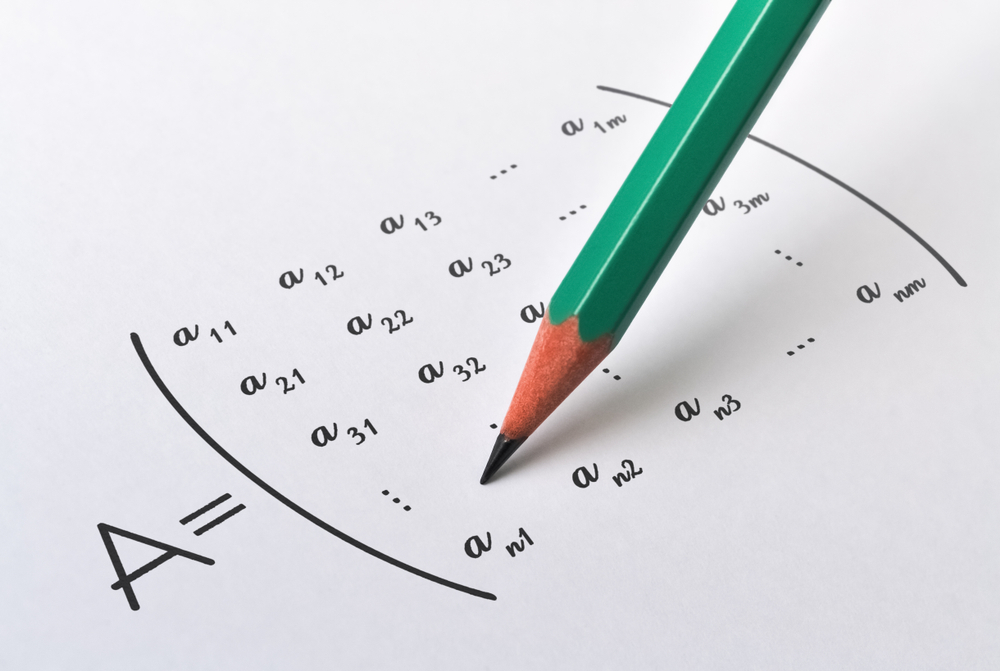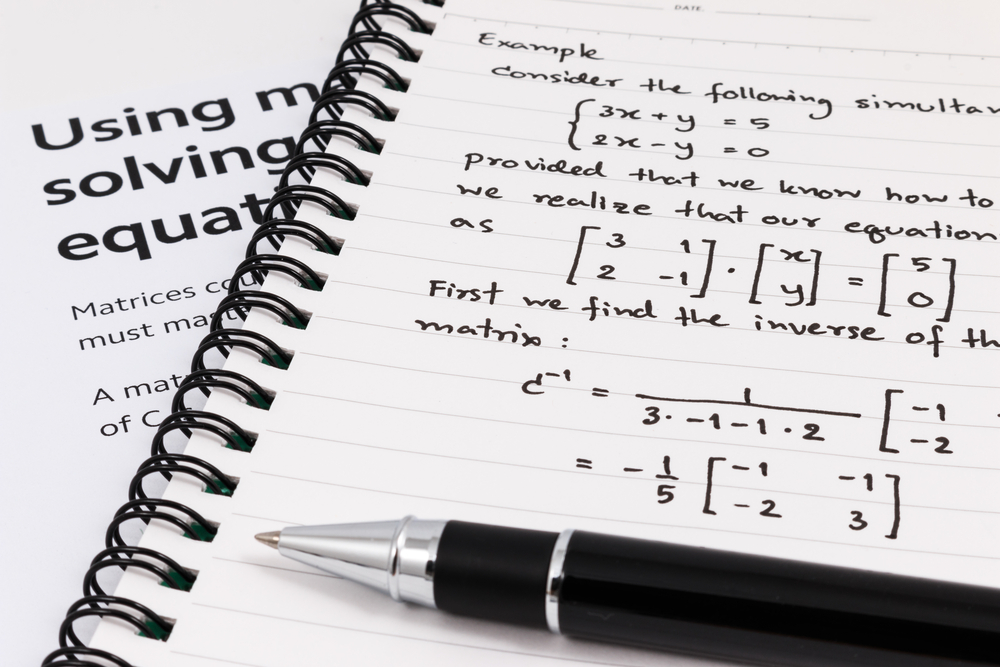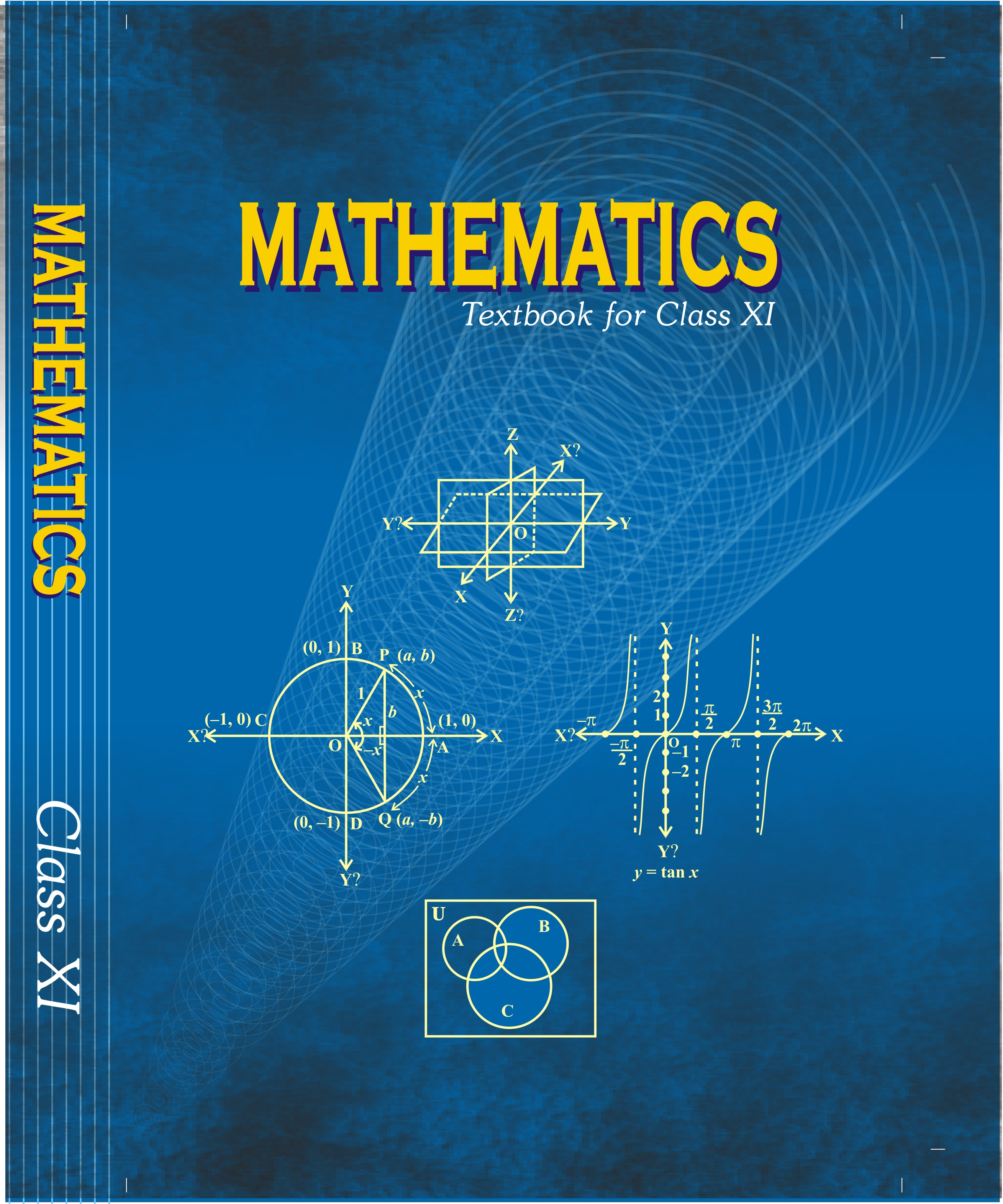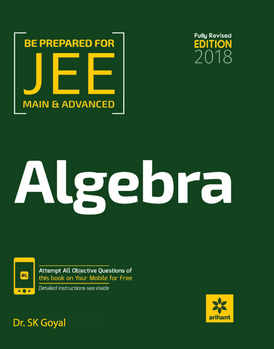# Matrices and Determinants   Share

## What is Matrices and Determinants

Matrices and Determinants:

In Mathematics, one of the interesting, easiest and important topic is Matrices and Determinants. Every year you will get at least 1 - 3 questions in JEE Main and other exams, directly and indirectly, the concept of this chapter will be involved in many other chapters, like integral and differential calculus. Concept of this chapter will be used for the axis-transformation concept. This chapter is totally new from the student point of view as you will see this chapter directly in 12th. So some students may find Matrices and Determinant little challenging to understand and solve problems initially. But as you solve more and more problems in this chapter, you will get familiar with concepts and chapter as a whole, then you will find that this is one of the easiest chapters. Afterward, the questions will appear easy for you. Matrices part may seem a little more difficult than Determinant but in the end, you will find both are easy to grasp,

## Why Matrices and Determinants:

Matrices and Determinant find a wide range of application in real-life problem, for example in adobe photoshop software matrix are used to process linear transformation to render images. A square matrix is used to represent a linear transformation of a geometric object. In computer programming matrices and its inverse are used for encrypting messages, to store data, perform queries and used as a data structure to solve algorithmic problems, etc. In robotics, the movement of the robot is programmed using a calculation based on matrices.## After studying this chapter :

1. It will be easy for you to understand the concept of the array in computer science (if u have taken computer science in +2).

2. It will be helping you to solve the problem involving simultaneous equation with as many unknown variables as equations.

3. Determinant will help you to solve problems related to areas and volume like the area of triangle and volume of a tetrahedron.

Prepare Online for JEE Main/NEET

Crack JEE 2021 with JEE/NEET Online Preparation Program

4. It will be helping you to organize your work in a much better way in the form of matrices and hence will help you to be clear in your mind in daily life.

5. And obviously, the chapter itself will help you to score some marks in the exam as it gets about 7% weight in jee main and around similar weight in other exams.

## Important Topics: Matrices and Determinants

1. Matrix and operation on matrices

2. Types of matrix

3. Transpose of a matrix, symmetric and skew-symmetric matrix

4. Conjugate of matrix, hermitian and skew-hermitian matrix

5. Determinant of matrix

6. Minor and cofactor of an element of matrix/determinant

7. Adjoint and inverse of a matrix

8. Elementary row operations and its use in finding the inverse of a matrix

9. System of linear equations and Cramer's rule

10. System of homogeneous linear equations

## Overview of the Matrix and Determinant:

Matrix: Set of numbers or objects or symbols represented in the form of the rectangular array is called a matrix. The order of the matrix is defined by the number of rows and number of columns present in the rectangular array of representation. For example

Matrix   has 2 rows and 3 columns so its order is said to be 2 × 3.

Any general element of the matrix is represented by ,  where represents the elements of the ith row and jth column.

Operations on matrices: Algebraic operation on matrices like addition, subtraction, multiplication, and division will be studied in one by one in the chapter in deep, which we will find that they are very easy to comprehend.

Transpose of the matrix: If A is a matrix then the matrix obtained by changing the columns of a matrix with rows or rows with columns is called the transpose of the matrix.

For example :

Conjugate of the matrix: If a matrix A has a complex number as it’s an element, then the matrix obtained by replacing those complex number by its conjugate is called conjugate of the matrix A and it is denoted by

The determinant of a matrix: a number which is calculated from the matrix. For determinant to exist, matrix A must be a square matrix. The determinant of a matrix is denoted by det A or |A|.

Minor and cofactor of an element in a matrix/determinant: Minor of any element  where i is the number of rows, j is the number of columns, is the det of matrix left over after deleting the ith row and jth column.

Adjoint of the matrix: transpose of the cofactor of the element of the matrix is known as the adjoint of the matrix.

The inverse of a Matrix: A non-singular square matrix “A” is said to be invertible if there exists a non-singular square matrix B such that AB = I=BA and the matrix B is called the inverse of the matrix A.## How to prepare Matrices and Determinants:

Matrices and Determinant is a topic useful in coordinate transformation and in some concept of differential equation as well as in the binomial theorem, you should be through with this chapter as well help some of those concepts as well as it will help you to score some easy marks in main exams.

1. Start with understanding basic concepts like Definition of the matrix, algebra of matrix, transpose of matrix, etc.

2. Then move ahead to the complex concept like adjoint of matrix and inverse of the matrix,

And then the system of equations, determinant, Cramer rules and homogenous equations.

3. After studying these concepts go through solved examples and then go to MCQ and practice the problem to make sure you understood the topic.

4. Solve the questions of the books which you are following and then go to previous year papers.

5. You can study matrix first then determinant or determinant first then matrix. In different books different order has been followed, you can choose your own order or the order of the coaching or material you are following.

6. While going through concept make sure you understand the derivation of formulas and try to derive them by your own, as many times you will not need the exact formula but some steps of derivation will be very helpful to solve the problem if you understand the derivation it will boost your speed in problem-solving.

7.Since this topic is heavily calculative practice as much as you can and while doing so remember the overall weight it has (7%).

8. At the end of chapter try to make your own short notes for quick revision, make a list of formula to revise quickly before exams or anytime when you required to revise the chapter, it will save lots of time for you.

## Best books for the preparation of this Complex number and Quadratic Equations:

First, finish all the concept, example and questions given in NCERT Maths Book. You must be thorough with the theory of NCERT. Then you can refer to the book Algebra, Arihant by Dr SK goyal or RD Sharma or Cengage Mathematics Algebra but make sure you follow any one of these not all. Matrix and determinant are explained very well in these books and there are an ample amount of questions with crystal clear concepts. Choice of reference book depends on person to person, find a book that suits you the best, depending on how well you are clear with the concepts and the difficulty of the questions you require.

## Maths Chapter-wise Notes for Engineering exams

 Chapters Chapters Name Chapter 1 Sets, Relations, and Functions Chapter 2 Complex Numbers and Quadratic Equations Chapter 4 Permutations and Combinations Chapter 5 Binomial Theorem and its Simple Applications Chapter 6 Sequence and Series Chapter 7 Limit, Continuity, and Differentiability Chapter 8 Integral Calculus Chapter 9 Differential Equations Chapter 10 Coordinate Geometry Chapter 11 Three Dimensional Geometry Chapter 12 Vector Algebra Chapter 13 Statistics and Probability Chapter 14 Trigonometry Chapter 15 Mathematical Reasoning Chapter 16 Mathematical Induction

### Topics from Matrices and Determinants

• Matrices, algebra of matrices, types of matrices ( AEEE, JEE Main, COMEDK UGET, KEAM ) (112 concepts)
• Adjoint and evaluation of inverse of a square matrix using deteminants and elementary transformations ( AEEE, JEE Main, COMEDK UGET, KEAM ) (28 concepts)
• Test of consistency and solution of simultaneous linear equation in two or three variables using determinants and matrices ( AEEE, JEE Main, COMEDK UGET, KEAM ) (30 concepts)
• Determinants and matrices of order two and three ( AEEE, JEE Main, COMEDK UGET, KEAM ) (4 concepts)
• Properties of determinants, evaluation of determinants ( AEEE, JEE Main, COMEDK UGET, KEAM ) (28 concepts)
• Matrices and Types of Matrices ( AEEE, JEE Main, COMEDK UGET, KEAM ) (8 concepts)
• Mathematical Operation on Matrices ( AEEE, JEE Main, COMEDK UGET, KEAM ) (10 concepts)
• Special Matrices and their Properties ( AEEE, JEE Main, COMEDK UGET, KEAM ) (22 concepts)
• Elementary Row Operations and Inverse of a Matrix by Elementary Row Operations ( AEEE, JEE Main, COMEDK UGET, KEAM ) (6 concepts)
• Determinant ( AEEE, JEE Main, COMEDK UGET, KEAM ) (4 concepts)
• Adjoint of Matrix ( AEEE, JEE Main, COMEDK UGET, KEAM ) (8 concepts)
• Inverse of a Matrix (Using Adjoint) ( AEEE, JEE Main, COMEDK UGET, KEAM ) (10 concepts)
• Multiplication of Determinant ( AEEE, JEE Main, COMEDK UGET, KEAM ) (2 concepts)
• Properties of Determinants ( AEEE, JEE Main, COMEDK UGET, KEAM ) (6 concepts)
• System of Simultaneous Linear equations ( AEEE, JEE Main, COMEDK UGET, KEAM ) (8 concepts)

### Important Books for Matrices and Determinants

••Exams
Articles
Questions